Ex 1.3

Chapter 1 Class 7 Integers
Serial order wise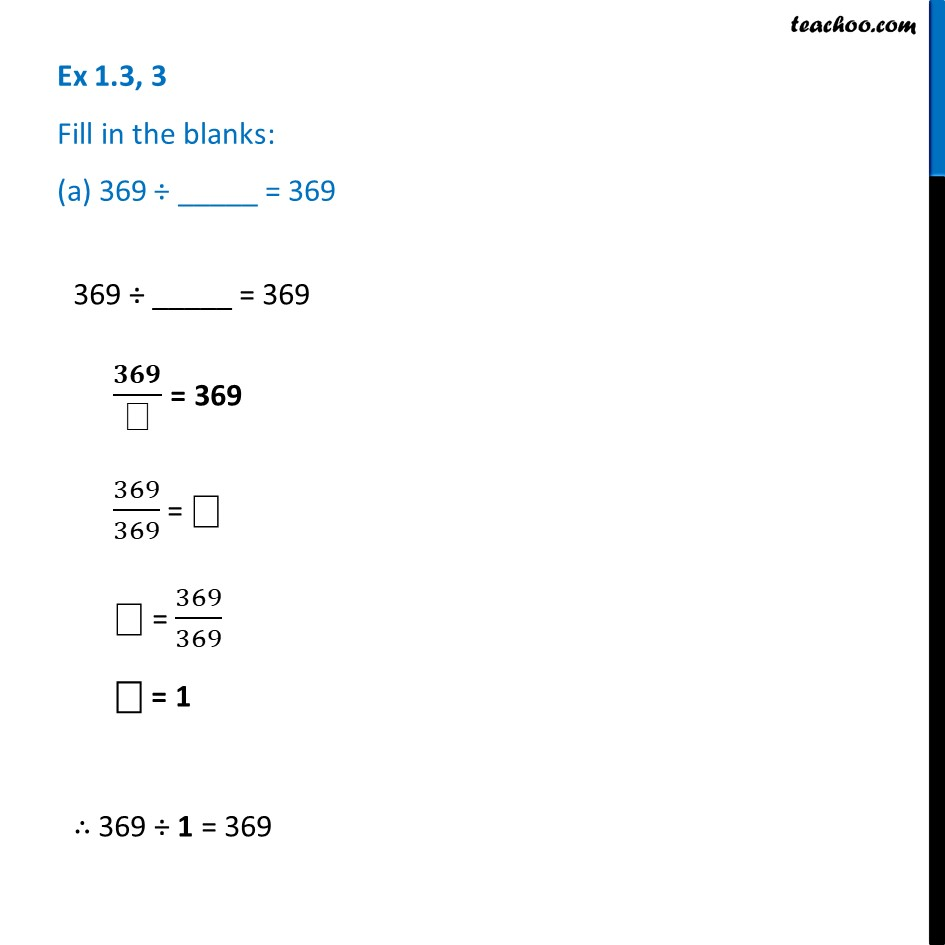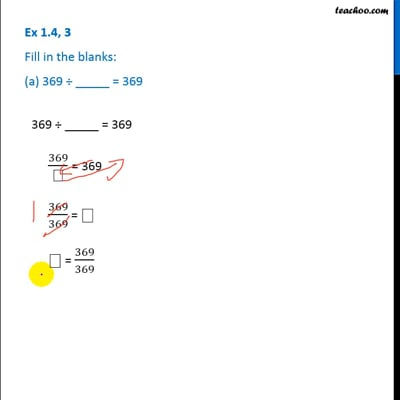This video is only available for Teachoo black users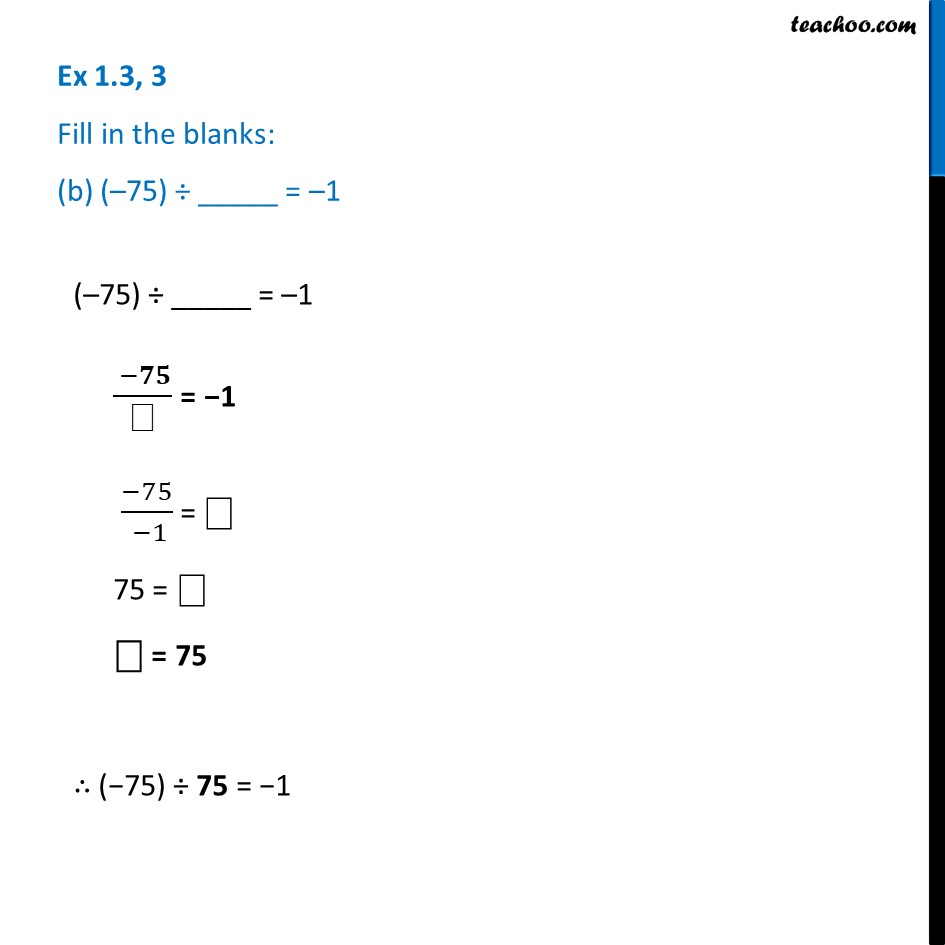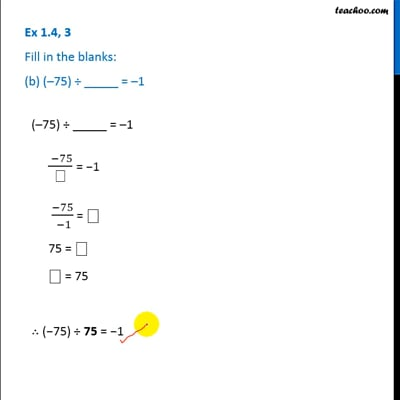This video is only available for Teachoo black users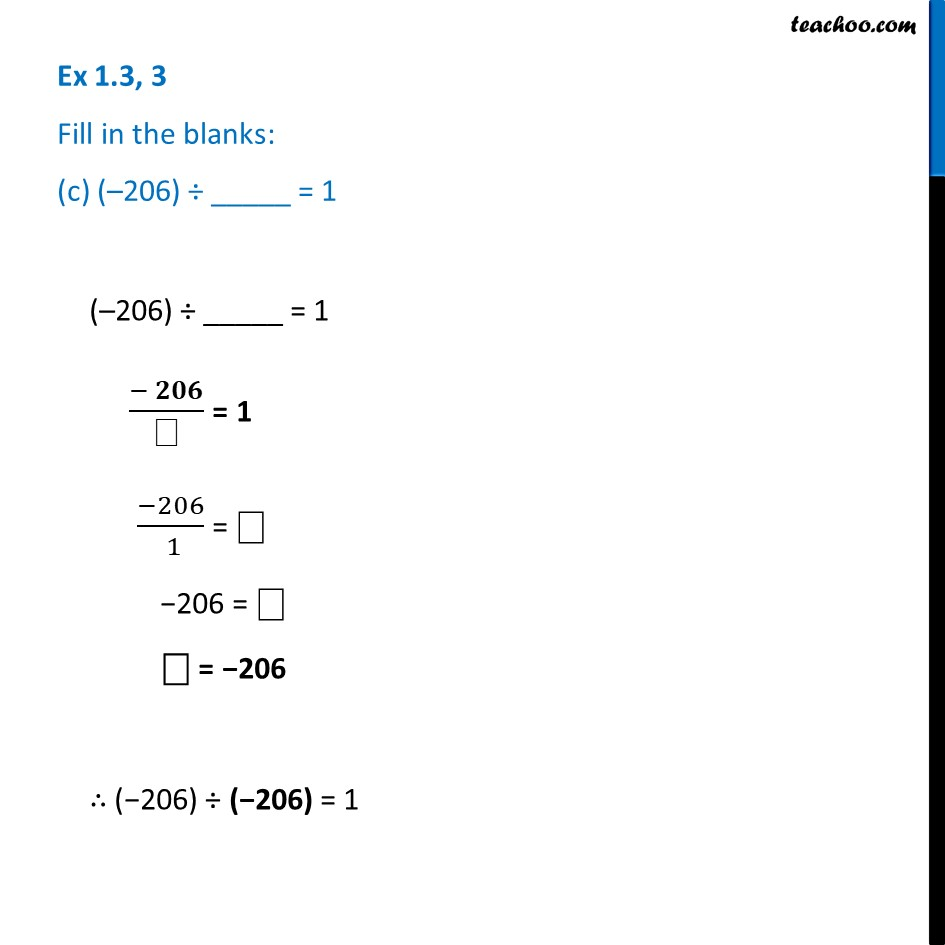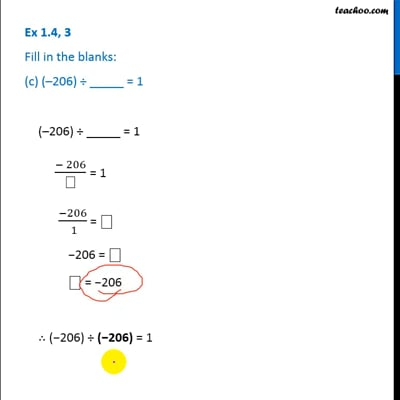This video is only available for Teachoo black users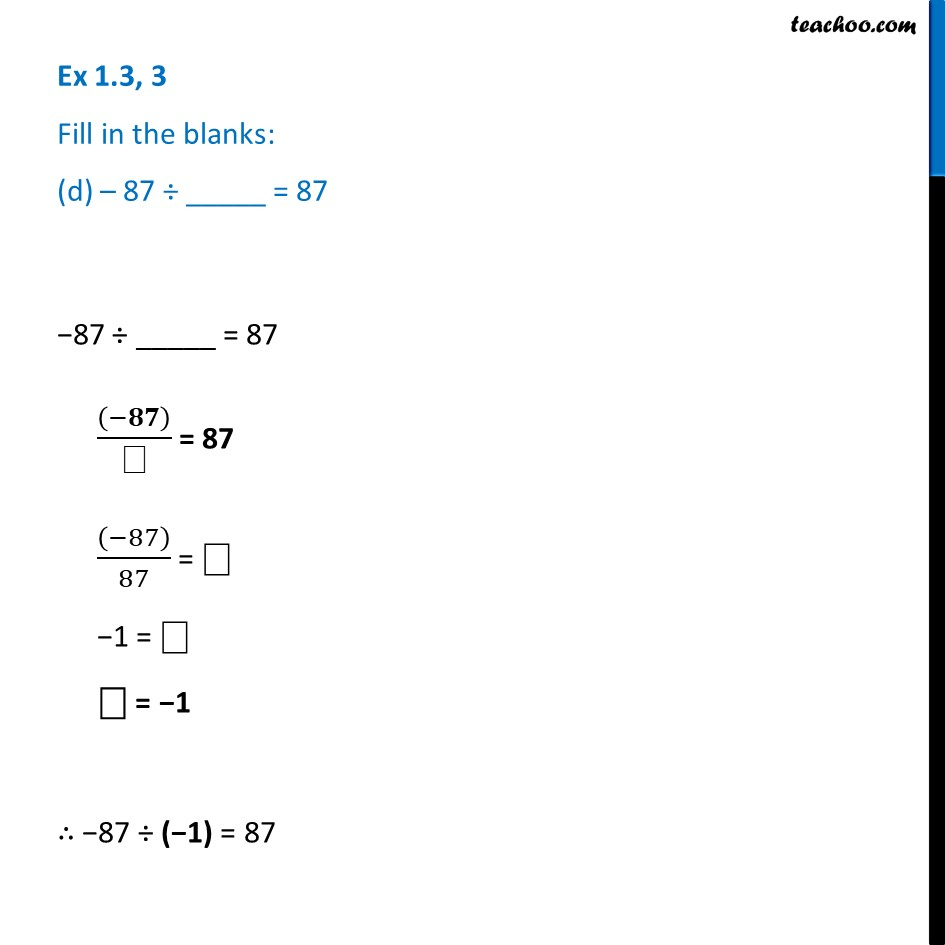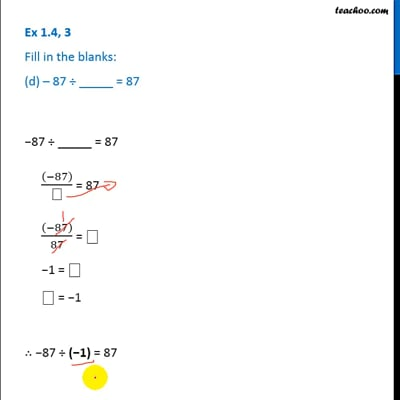This video is only available for Teachoo black users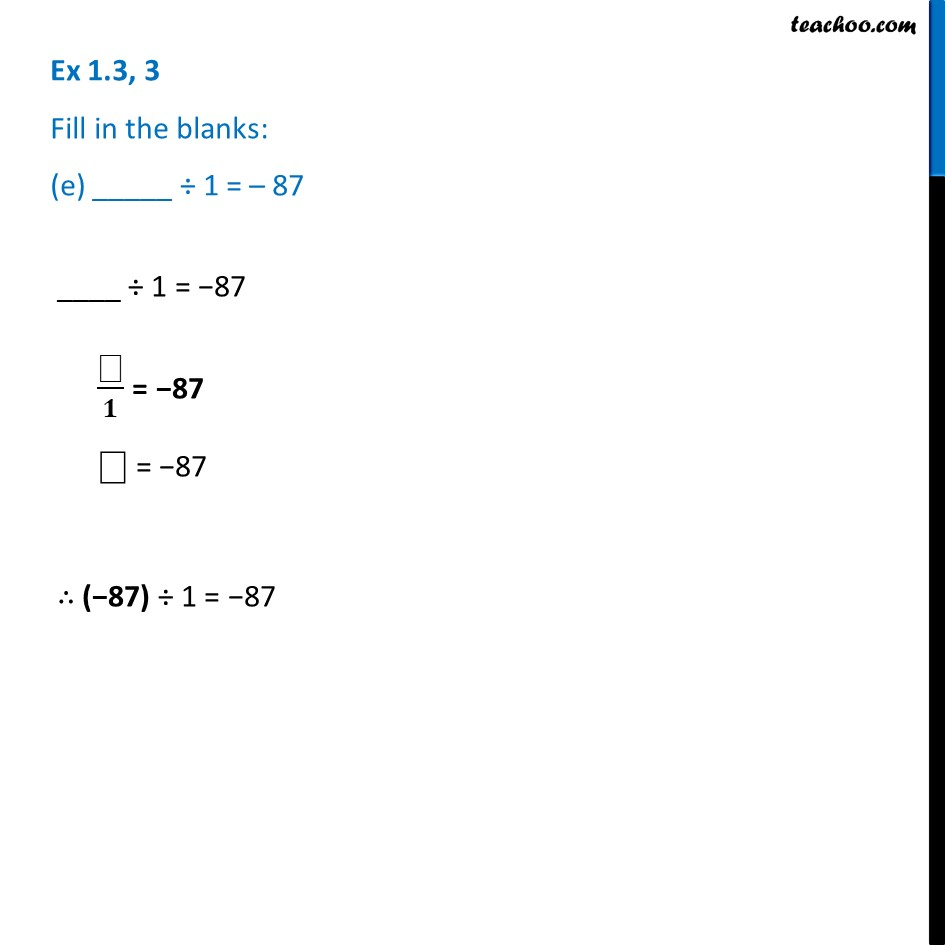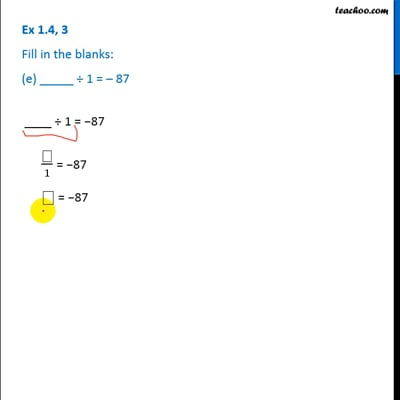This video is only available for Teachoo black users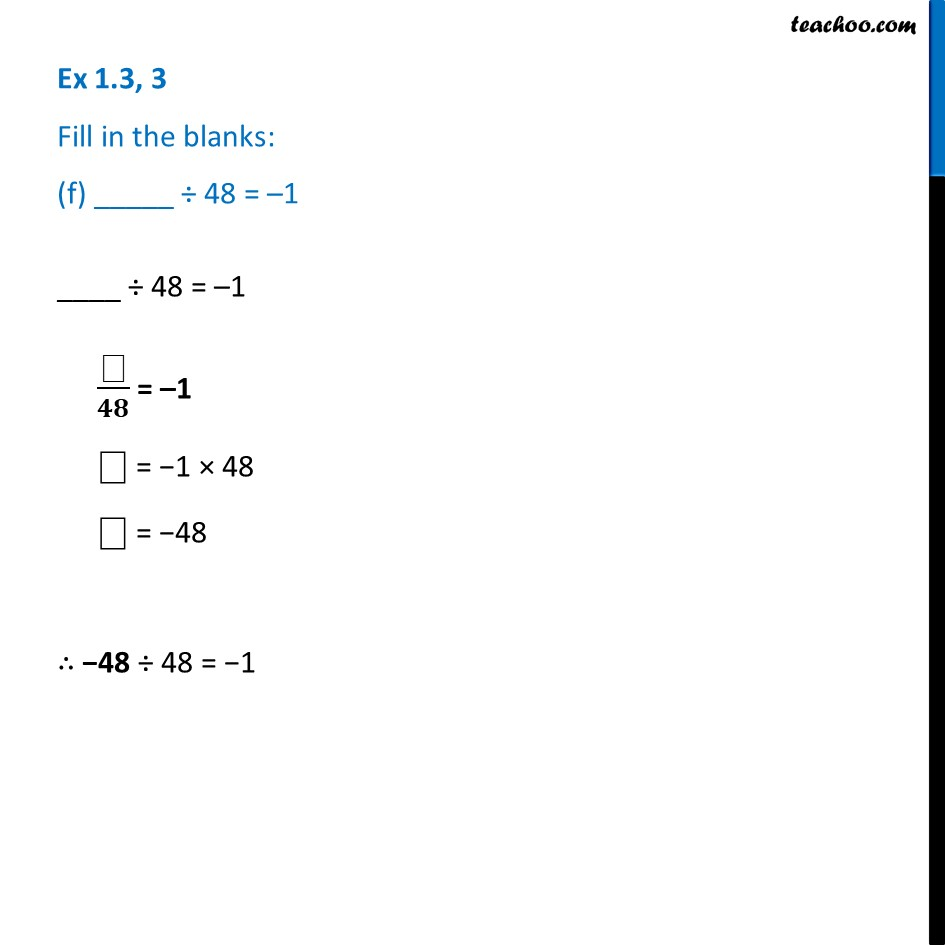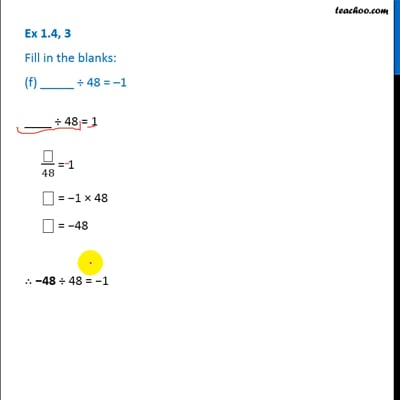This video is only available for Teachoo black users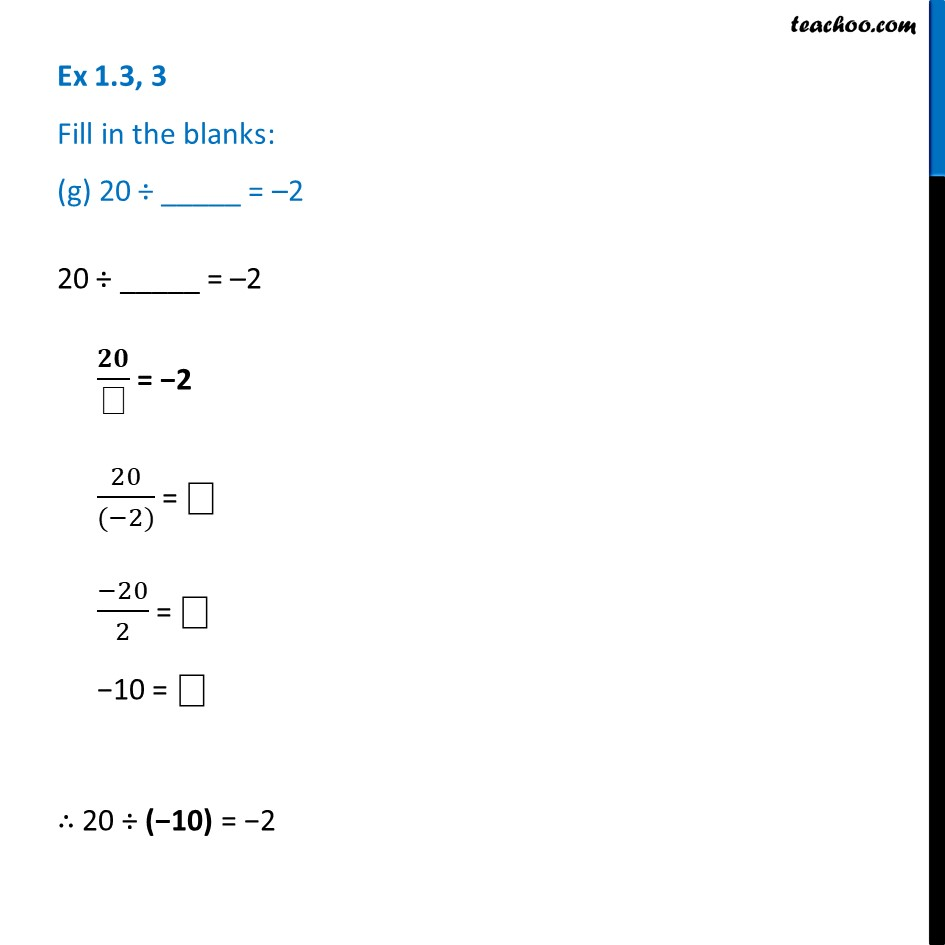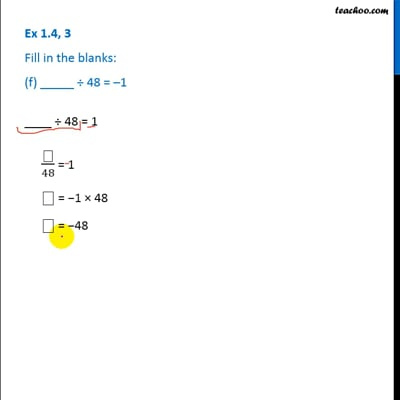This video is only available for Teachoo black users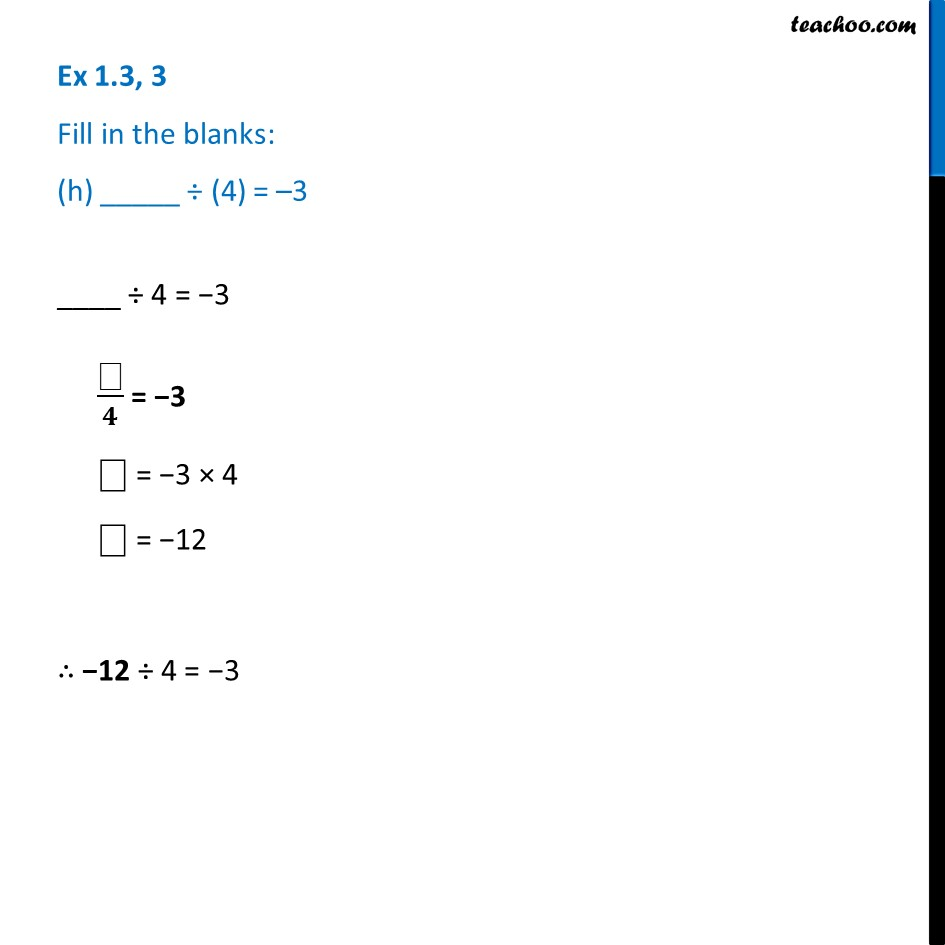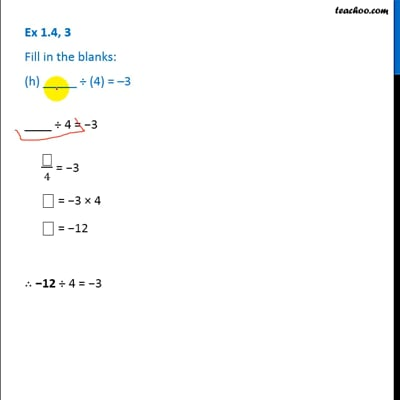This video is only available for Teachoo black users

Learn in your speed, with individual attention - Teachoo Maths 1-on-1 Class

### Transcript

Ex 1.3, 3 Fill in the blanks: (a) 369 ÷ _____ = 369 369 ÷ _____ = 369 𝟑𝟔𝟗/⎕ = 369 369/369 = ⎕ ⎕ = 369/369 ⎕ = 1 ∴ 369 ÷ 1 = 369 Ex 1.3, 3 Fill in the blanks: (b) (–75) ÷ _____ = –1(–75) ÷ _____ = –1 ( −𝟕𝟓)/⎕ = −1 (−75)/( −1) = ⎕ 75 = ⎕ ⎕ = 75 ∴ (−75) ÷ 75 = −1 Ex 1.3, 3 Fill in the blanks: (c) (–206) ÷ _____ = 1 (–206) ÷ _____ = 1 (− 𝟐𝟎𝟔)/⎕ = 1 (−206)/( 1) = ⎕ −206 = ⎕ ⎕ = −206 ∴ (−206) ÷ (−206) = 1 Ex 1.3, 3 Fill in the blanks: (d) – 87 ÷ _____ = 87−87 ÷ _____ = 87 ((−𝟖𝟕))/⎕ = 87 ((−87))/87 = ⎕ −1 = ⎕ ⎕ = −1 ∴ −87 ÷ (−1) = 87 Ex 1.3, 3 Fill in the blanks: (e) _____ ÷ 1 = – 87____ ÷ 1 = −87 ⎕/𝟏 = −87 ⎕ = −87 ∴ (−87) ÷ 1 = −87 Ex 1.3, 3 Fill in the blanks: (f) _____ ÷ 48 = –1____ ÷ 48 = –1 ⎕/𝟒𝟖 = –1 ⎕ = −1 × 48 ⎕ = −48 ∴ −48 ÷ 48 = −1 Ex 1.3, 3 Fill in the blanks: (g) 20 ÷ _____ = –220 ÷ _____ = –2 𝟐𝟎/⎕ = −2 20/((−2)) = ⎕ (−20)/2 = ⎕ −10 = ⎕ ∴ 20 ÷ (−10) = −2 Ex 1.3, 3 Fill in the blanks: (h) _____ ÷ (4) = –3____ ÷ 4 = −3 ⎕/𝟒 = −3 ⎕ = −3 × 4 ⎕ = −12 ∴ −12 ÷ 4 = −3